Posted on

MS Excel Shortcuts

Selection

Select entire row

To select all the cells in a specific row you can use the command Shift + Spacebar.

Select entire column

To select a particular column, use the command Ctrl + Spacebar.Select entire worksheet

To select all the cells in a worksheet, use the Ctrl + A command.

You can add cells adjacent to an existing selection by holding down the Shift key and clicking on them.

Extend selection one cell to the right

To select cells to the right of the current cell you can use the Shift + right arrow key commands. This will select the cell immediately to the right of the current cell.Extend selection by one cell to the left

Just as you can select a cell to the right you can also select one to the left of your current cell. The command Shift + left arrow will allow you to select a cell to the left of the cell you are currently on.

Extend by one cell up

To select a cell that is directly above the current cell, use the command Shift + up arrow.Extend by one cell down

Similarly, use the command Shift + down arrow to select a cell below the current cell.

Extend to the last cell on the right

Ctrl + Shift + right arrow is used to select the last cell to the right of the current cell.

Extend to the last cell on the left

To select the cell on the extreme left of the current cell, use the command Ctrl + Shift + left arrow.

Extend to the last cell up

Similarly, Ctrl + Shift + up arrow can be used to select up to the last cell on the top.

Extend to the last cell down

Ctrl + Shift + down arrow will allow you to select all the cells till the last cell below the current cell.

Extend selection to start of row

Shift + Home is the command used to extend the selection up to the beginning of a particular row.

Extend selection to first cell

To extend the selection from the current cell to the very first cell, use the command Ctrl + Shift + Home.

Extend selection to last cell

Ctrl + Shift + End is the command used to select cells up to the very last cell of the worksheet.

To select specific cells, such as those that have comments, use the command Ctrl + Shift + O. Only the cells that have comments attached to them will get highlighted.

Select one character right

Shift + right arrow is used to select a single character to the right of the current character.

Select one character left

To select a single character to the left of the current character use the command Shift + left arrow key.Select one word to the right

Use Ctrl + Shift + right arrow to select a word to the right of the present word.

Select one word to the left

Use Ctrl + Shift + left arrow to select a word to the left of the present word.Posted on

Data Entry & Management in Excel

One of the main uses of Microsoft Excel is for data entry, maintenance and monitoring. Data in Excel can be organized in columns, as a table, or in a pie chart or graph.Right now, we’ll be focusing on the basics of data entry and management in a worksheet. Data here is entered in a table that consists of rows and columns.

The top rows of tables have headings that go down to the left and are used to identify the data being entered in the tables. This lets Excel do any necessary calculations through the use of formulas. The program definitely makes it easier to manage and process data.To make it even easier, Excel also provides a number of ways to formatting the data. If you want to locate and review some information, you’ll appreciate these data formatting features. They can be used for data formatting in individual cells, different columns and rows, and for formatting entire tables.

In previous versions of Excel each worksheet contained a billion cells, while the most recent updates released by Microsoft have almost seventeen billion cells.

To make it easier to access and reference these multitudinous cells, each of them has been given an address. This is called a cell reference.Posted on

What is Excel Reference?

Cell references in Excel are very important. Understand the difference between relative, absolute and mixed reference, and you are on your way to success.Relative Reference

By default, Excel uses relative reference. See the formula in cell D2 below. Cell D2 references (points to) cell B2 and cell C2. Both references are relative.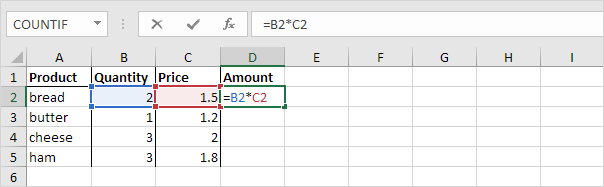1. Select cell D2, click on the lower right corner of cell D2 and drag it down to cell D5.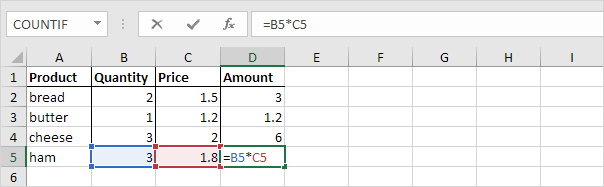Cell D3 references cell B3 and cell C3. Cell D4 references cell B4 and cell C4. Cell D5 references cell B5 and cell C5. In other words: each cell references its two neighbors on the left.

Absolute Reference

See the formula in cell E3 below.1. To create an absolute reference to cell H3, place a \$ symbol in front of the column letter and row number of cell H3 (\$H\$3) in the formula of cell E3.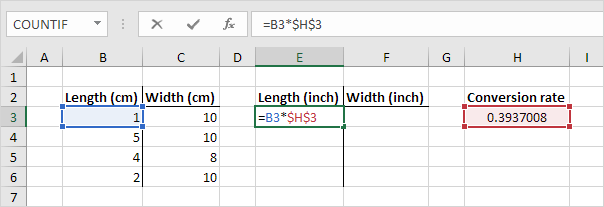2. Now we can quickly drag this formula to the other cells.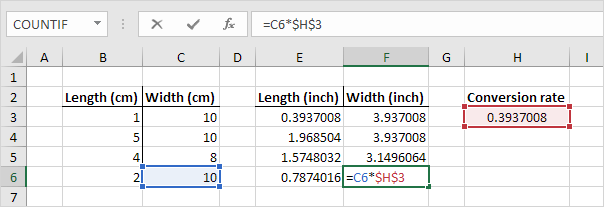The reference to cell H3 is fixed (when we drag the formula down and across). As a result, the correct lengths and widths in inches are calculated.

Mixed Reference

Sometimes we need a combination of relative and absolute reference (mixed reference).

1. See the formula in cell F2 below.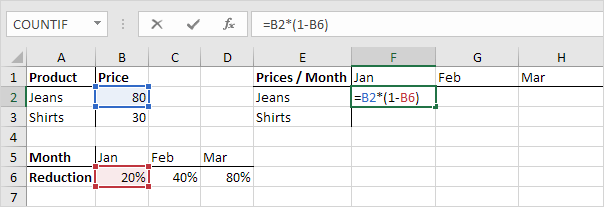2. We want to copy this formula to the other cells quickly. Drag cell F2 across one cell, and look at the formula in cell G2.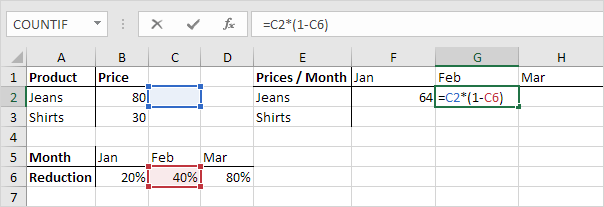Do you see what happens? The reference to the price should be a fixed reference to column B. Solution: place a \$ symbol in front of the column letter of cell B2 (\$B2) in the formula of cell F2. In a similar way, when we drag cell F2 down, the reference to the reduction should be a fixed reference to row 6. Solution: place a \$ symbol in front of the row number of cell B6 (B\$6) in the formula of cell F2.

Result:Note: we don’t place a \$ symbol in front of the row number of B2 (this way we allow the reference to change from B2 (Jeans) to B3 (Shirts) when we drag the formula down). In a similar way, we don’t place a \$ symbol in front of the column letter of B6 (this way we allow the reference to change from B6 (Jan) to C6 (Feb) and D6 (Mar) when we drag the formula across).

3. Now we can quickly drag this formula to the other cells.Posted on

MS Excel Index Function

The Excel INDEX function returns the value at a given position in a range or array. You can use index to retrieve individual values or entire rows and columns. INDEX is often used with the MATCH function, where MATCH locates and feeds a position to INDEX.Syntax

=INDEX (array, row_num, [col_num], [area_num])

=INDEX (reference, row_num, [col_num], [area_num])

Arguments

• array – A range of cells, or an array constant.
• row_num – The row position in the reference or array.
• col_num – [optional] The column position in the reference or array.
• area_num – [optional] The range in reference that should be used.Explanation:

1.We want to know how much student 5 got In physics. So we first type index and select array (all the numbers).

2.Forrow_num we can give 5 or use match function (it is easier to use match function when the list is long).

3. For column_num we can give 4 or use match function (it is easier to use match function when there are many column).

Usage notes

Use the INDEX function to get a value from a list or table based on its location. For example, the formula =INDEX(A1:B5,2,2) will return the value at the address B2.

The INDEX function has two forms: array and reference.Posted on

MS Excel Sumproduct Function

You have to install MS Office first. Please click the link below:Suppose you bought 4 products in many quantities. This is time consuming to multiply unit with price and the add them together. Excel has a built in formula for that.

The SUMPRODUCT function multiplies ranges or arrays together and returns the sum of products.SUMPRODUCT is an incredibly versatile function that can be used to count and sum like COUNTIFS or SUMIFS, but with more flexibility. Other functions can easily be used inside SUMPRODUCT to extend functionality even further.

Syntax
=SUMPRODUCT (array1, [array2], …)
Arguments
• array1 – The first array or range to multiply, then add.
• array2 – [optional] The second array or range to multiply, then add.

Select array 1 then multiply with selected array2 and press enter.

How to do this calculation manually:
(3*2) +(5*1.50) +(1*60) +(2*10.5) =94.5

Usage notes

The SUMPRODUCT function works with arrays, but it doesn’t require the normal array syntax (Ctrl + Shift + Enter) to enter. The purpose of the SUMPRODUCT function is to multiply, then sum, arrays. If only one array is supplied, SUMPRODUCT will simply sum the items in the array. Up to 30 arrays can be supplied.Posted on

MS Excel Sum Function

We all need to pay bills. Suppose you use 10 utilities (rent, electricity, Wi-Fi etc.). And you need to pay bill for that. And now you want to know how much did you spend on that per month. Here excel will save your time. You don’t need to input the numbers 10 times in a calculator. You can use spreadsheets to get it done quickly!The Excel SUM function returns the sum of values supplied as multiple arguments. SUM can handle up to 255 individual arguments, which can include numbers, cell references, ranges, arrays, and constants.

Syntax

=SUM (number1, [number2], [number3], …)

Arguments

• number1– The first item to sum.
• number2 – [optional] The second item to sum.
• number3– [optional] The third item to sum.

Usage notes

1. Select the numbers you want to add. You can do it individually by clicking each cell or drag them with mouse from top to bottom (shown in this pic).
2. Hit Enter and you will see the sum.

The keyboard shortcut for sum is (Alt) + (=)

There are also other powerful Excel functions, including MS Excel LookUp function.

You can get all the 50 most powerful MS Excel functions. Click the link below:However, firstly you have to install MS Office programs, which includes MS Excel. Please click the link below to get the software.Posted on

MS Excel LookUp Function

The Microsoft Excel LOOKUP function is one of the most powerful functions of MS Excel. The function helps in finding a value from a range/table or from a vector/(column, row).

You can use this function to get values from the same spreadsheet and workbook or from different spreadsheets/workbooks.LOOKUP function is searching specific values within a data set. If you want to be an excel expert then you have to be a great knowledge about LOOKUP formula. It will also save you lots of time.

Here you get a list of most popular excel LOOKUP formulas.

OFFSET MATCH MATCH – The offset function in Excel is an exceptionally flexible formula that, when joined with the Match formula, can imitate the usefulness of a lookup. The Match formula at that point enables you to move vertically and horizontally from that reference indicate discovers your arrival value. The key difference in utilizing the offset function is that you should begin your formula with a solitary cell reference, regularly the upper left hand corner of your information run.

VLOOKUP MATCH – By joining the VLOOKUP and Match elements of Excel, the initially static column reference inside the VLOOKUP equation winds up plainly unique, now at whatever point you embed a column into your informational index, your column reference will refresh with the goal that despite everything you have a similar return value.
INDEX MATCH – The syntax for composing an Index Match can be difficult at first to recall. However, after regular use, it is truly not a difficult formula to remember; I currently use Index Match almost only for the greater part of my lookups.

INDEX MATCH MATCH – Adding an extra Match formula to the basic Index Match formula improves it with the goal that you can play out a matrix lookup. It is an indistinguishable formula from Index Match, with the exception of your column reference is currently powerful.

VLOOKUP – Full abbreviation of VLOOPKUP is Vertical LOOKUP. This VLOOKUP is the most famous and oldest LOOKUP formula in excel. In your excel learning it will be a new milestone when you write a VLOOUP formulas in a first time. By the way, if you use it continually then you will find the flow of this task.

HLOOKUP – Full abbreviation of HLOOPKUP is Horizontal LOOKUP. HLOOKUP works similarly in VLOOKUP. VLOOKUP will find values vertically in columns and HLOOKUP will find values horizontally in rows. VLOOKUP and HLOOKUP’s syntaxes are same. Main difference with the VLOOKUP is that you must horizontal range in HLOOKKUP function.

VLOOKUP HLOOKUP – A matrix lookup infers that you are looking into both a vertical and horizontal value to pinpoint the arrival value you need to pull. When you consolidate Excel’s basic vertical and horizontal lookup formulas, you wind up with a grid query.

You can install MS products, click the link below: